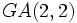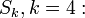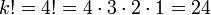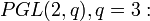# Difference between revisions of "Symmetric group:S4"

View a complete list of particular groups (this is a very huge list!)[SHOW MORE]

## Definition

### Verbal definition

The symmetric group$S_4$ or$\operatorname{Sym}(4)$, also termed the symmetric group of degree four, is defined in the following equivalent ways:$\langle s_1, s_2, s_3 \mid s_1^2 = s_2^2 = s_3^2 = e, (s_1s_2)^3 = (s_2s_3)^3 = (s_1s_3)^2 = e \rangle$.

where$e$ is the identity element.

In particular, it is a Coxeter group.

• The full tetrahedral group: The group of all (not necessarily orientation-preserving) symmetries of the regular tetrahedron. This is denoted as$T_h$.
• The von Dyck group with parameters$(4,3,2)$. In other words, it has the presentation:$\langle a,b,c \mid a^4 = b^3 = c^2 = abc = e \rangle$.

• The octahedral group or cube group: group of orientation-preserving symmetries of the cube (or equivalently, the octahedron). This is denoted as$O$.
• The projective general linear group of degree two over the field of three elements:$PGL(2,3)$.
• The general affine group of degree two over the field of two elements:$GA(2,2)$.

## Families

Generic name for family member Definition Parametrization of family Parameter value(s) for this member Other members Comments
symmetric group on finite set group of all permutations on a finite set by a nonnegative integer, denoting size of set acted on 4 click here for a list
Coxeter group has a presentation of a particular form Coxeter matrix describing the presentation click here for a list symmetric groups on finite sets are Coxeter groups
von Dyck group$\langle a,b,c \mid a^p = b^q = c^r = abc = e \rangle$$(p,q,r)$ (4,3,2) click here for a list$S_4$ is a spherical von Dyck group, i.e., it occurs as a finite subgroup of$SO(3,\R)$. In particular, this makes it a Coxeter group. Further information: Classification of finite subgroups of SO(3,R)
triangle group$\langle s_1,s_2,s_3 \mid s_1^2 = s_2^2 = s_3^2 = (s_1s_2)^p = (s_2s_3)^q = (s_3s_1)^r = e \rangle$$(p,q,r)$ (3,3,2) click here for a list$S_4$ is a spherical triangle group
projective general linear group projective general linear group of given degree over a given field name of field, degree field:F3 (size three), degree two

## Arithmetic functions

Want to compare and contrast arithmetic function values with other groups of the same order? Check out groups of order 24#Arithmetic functions

### Single-valued functions

Function Value Similar groups Explanation for function value
order (number of elements, equivalently, cardinality or size of underlying set) 24 groups with same order As$\! S_k, k = 4:$$\! k! = 4! = 4 \cdot 3 \cdot 2 \cdot 1 = 24$

as$\! PGL(2,q), q = 3:$$\! (q^2 - 1)(q^2 - q)/(q - 1) = (3^2 - 1)(3^2 - 3)/2 = 24$

As$\! GA(2,q), q = 2:$$\! q^2 \cdot (q^2 - 1) \cdot (q^2 - q) = 2^2 \cdot (2^2 - 1)(2^2 - 2) = 4 \cdot 3 \cdot 2 = 24$
exponent 12 groups with same order and exponent | groups with same exponent elements of order$2,3,4$, their lcm is$12$
derived length 3 groups with same order and derived length | groups with same derived length Derived series goes through alternating group:A4 and Klein four-group of double transpositions.
nilpotency class -- not a nilpotent group.
Frattini length 1 groups with same order and Frattini length | groups with same Frattini length Frattini-free group: intersection of maximal subgroups is trivial.
minimum size of generating set 2 groups with same order and minimum size of generating set | groups with same minimum size of generating set$(1,2), (1,2,3,4)$; see also symmetric group on a finite set is 2-generated
subgroup rank of a group 2 groups with same order and subgroup rank of a group | groups with same subgroup rank of a group All proper subgroups are cyclic or have generating set of size two (D8, Klein four-group, and A4)
max-length of a group 4 groups with same order and max-length of a group | groups with same max-length of a group Series for Sylow 2-subgroup followed by whole group, OR derived series refined at end.
composition length 4 groups with same order and composition length | groups with same composition length
chief length 3 groups with same order and chief length | groups with same chief length
number of subgroups 30 groups with same order and number of subgroups | groups with same number of subgroups
number of conjugacy classes 5 groups with same order and number of conjugacy classes | groups with same number of conjugacy classes as$S_k, k =4:$ the number of conjugacy classes is$\! p(k) = p(4) = 5$, where$p$ is the number of unordered integer partitions; see cycle type determines conjugacy class

as$PGL(2,q), q = 3:$ the number of conjugacy classes is$q + 2 = 3 + 2 = 5$; see projective general linear group of degree two

as$GA(2,q), q = 2:$$\! q^2 + 1 = 2^2 + 1 = 5$
number of conjugacy classes of subgroups 11 groups with same order and number of conjugacy classes of subgroups | groups with same number of conjugacy classes of subgroups

### Lists of numerical invariants

List Value Explanation/comment
conjugacy class sizes$1,3,6,6,8$ See element structure of symmetric group:S4, element structure of symmetric groups, cycle type determines conjugacy class
order statistics$1 \mapsto 1, 2 \mapsto 9, 3 \mapsto 8, 4 \mapsto 6$
degrees of irreducible representations$1,1,2,3,3$ See linear representation theory of symmetric group:S4, linear representation theory of symmetric groups

## Group properties

COMPARE AND CONTRAST: Want to know more about how this group compares with symmetric groups of other degrees? Read contrasting symmetric groups of various degrees.
Property Satisfied Explanation Comment
Abelian group No$(1,2)$,$(1,3)$ don't commute$S_n$ is non-abelian,$n \ge 3$.
Nilpotent group No Centerless: The center is trivial$S_n$ is non-nilpotent,$n \ge 3$.
Metacyclic group No No cyclic normal subgroup$S_n$ is not metacyclic,$n \ge 4$.
Supersolvable group No No cyclic normal subgroup$S_n$ is not supersolvable,$n \ge 4$.
Solvable group Yes Length three, commutator subgroup is$A_4$, its commutator is Klein four-group Largest$n$ for which$S_n$ is solvable.
T-group No Double transposition generates non-normal 2-subnormal subgroup Only$n$ for which$S_n$ isn't a T-group.
HN-group No Double transposition generates subnormal non-hypernormalized subgroup Only$n$ for which$S_n$ isn't hypernormalized.
Complete group Yes Centerless and every automorphism's inner Symmetric groups are complete except the ones of degree$2,6$.
Monolithic group Yes Monolith is the Klein four-group of double transpositions All symmetric groups are monolithic;$n=4$ is the only case the monolith is not the alternating group.
One-headed group Yes The alternating group is the unique maximal normal subgroup True for all$n > 1$.
Group having subgroups of all orders dividing the group order Yes (See subgroup list) Largest$n$ for which this is true.
Rational-representation group Yes Symmetric groups are rational-representation
Rational group Yes Symmetric groups are rational Also see classification of rational dihedral groups
Ambivalent group Yes Symmetric groups are ambivalent

## Endomorphisms

### Automorphisms

Since$S_4$ is a complete group, it is isomorphic to its automorphism group, where each element of$S_4$ acts on$S_4$ by conjugation. In fact, for$n \ne 2,6$, the symmetric group$S_n$ is a complete group. Further information: Symmetric groups on finite sets are complete

### Endomorphisms$S_4$ admits four kinds of endomorphisms (that is, it admits more endomorphisms, but any endomorphism is equivalent via an automorphism to one of these four):

• The endomorphism to the trivial group
• The identity map
• The retraction to a group of order two, given by the sign homomorphism.
• The retraction to a symmetric group on three of the elements, with kernel being the Klein four-group comprising the identity element and the double transpositions. (Note that all such retractions are equivalent, and there are other equivalent endomorphisms obtained by composing such a retraction with an automorphism).

## Elements

Further information: element structure of symmetric group:S4

### Conjugacy class structure

There are five conjugacy classes, corresponding to the cycle types, because cycle type determines conjugacy class. Further, each cycle type corresponds to a partition of$4$.

### Automorphism class structure

Since$S_4$ is a complete group, all its automorphisms are inner automorphisms, and, in particular, the classification of elements up to conjugacy is the same as the classification up to automorphisms.

## Subgroups

Further information: Subgroup structure of symmetric group:S4

Here is a complete list of subgroups. Note that since$S_4$ is a complete group, every automorphism is inner, so the classification of subgroups upto conjugacy is equivalent to the classification of subgroups upto automorphism.

1. The trivial subgroup. Isomorphic to trivial group.(1)
2. The two-element subgroup generated by a transposition, such as$(1,2)$. Isomorphic to cyclic group of order two.(6)
3. The two-element subgroup generated by a double transposition, such as$(1,2)(3,4)$. Isomorphic to cyclic group of order two. (3)
4. The four-element subgroup generated by two disjoint transpositions, such as$\langle (1,2) \ , \ (3,4) \rangle$. Isomorphic to Klein four-group. (3)
5. The unique four-element subgroup comprising the identity and the three double transpositions. Isomorphic to Klein four-group. (1)
6. The four-element subgroup spanned by a 4-cycle. Isomorphic to cyclic group of order four.(3)
7. The eight-element subgroup spanned by a 4-cycle and a transposition that conjugates this cycle to its inverse. Isomorphic to dihedral group of order eight. (3)
8. The three-element subgroup spanned by a three-cycle. Isomorphic to cyclic group of order three.(4)
9. The six-element subgroup comprising all permutations that fix one element. Isomorphic to symmetric group on three elements. (4)
10. The alternating group: the subgroup of all even permutations. Isomorphic to alternating group:A4.(1)
11. The whole group.(1)

### Normal subgroups

The only normal subgroups of$S_4$ are: the trivial subgroup (type (1)), the Klein four-group (type (5) -- note that type (4) are non-normal subgroups), the alternating group$A_4$ (type (10)), and the whole group (type (11)). It turns out that the characteristic subgroups, the fully characteristic subgroups, the permutable subgroups, and the retraction-invariant subgroups all coincide with the normal subgroups.

### Sylow subgroups

The Sylow subgroups are as follows:

• The$2$-Sylow subgroups are dihedral groups of order eight, type (7) in the list.
• The$3$-Sylow subgroups are cyclic groups of order three, type (9) in the list.

Further information: Subgroup structure of symmetric group:S4

## Subgroup-defining functions

Subgroup-defining function Subgroup type in list Isomorphism class Comment
Center (1) Trivial group The group is centerless
Commutator subgroup (10) Alternating group:A4
Frattini subgroup (1) Trivial group The normal cores of the maximal subgroups of orders$6$ and$8$ intersect trivially.
Socle (5) Klein four-group This subgroup is the unique minimal normal subgroup, i.e., the monolith, and the group is monolithic.

## Supergroups

The symmetric group$S_4$ is contained in higher symmetric groups, most notably the symmetric group on five elements$S_5$.

## Extensions

These include$GL(2,3)$ whose inner automorphism group is$S_4$ (specifically$S_4$ is the quotient of$GL(2,3)$ by its scalar matrices).

## Implementation in GAP

### Group ID

This finite group has order 24 and has ID 12 among the groups of order 24 in GAP's SmallGroup library. For context, there are 15 groups of order 24. It can thus be defined using GAP's SmallGroup function as:

SmallGroup(24,12)

For instance, we can use the following assignment in GAP to create the group and name it$G$:

gap> G := SmallGroup(24,12);

Conversely, to check whether a given group$G$ is in fact the group we want, we can use GAP's IdGroup function:

IdGroup(G) = [24,12]

or just do:

IdGroup(G)

to have GAP output the group ID, that we can then compare to what we want.

### Short descriptions

Description Functions used Mathematical comment
SymmetricGroup(4) SymmetricGroup symmetric group of degree four (acting by default on$\{ 1,2,3,4 \}$. We can also specify a set on which we make the group act, e.g., SymmetricGroup([1,2,4,8])
PGL(2,3) PGL projective general linear group of degree two over the field of three elements